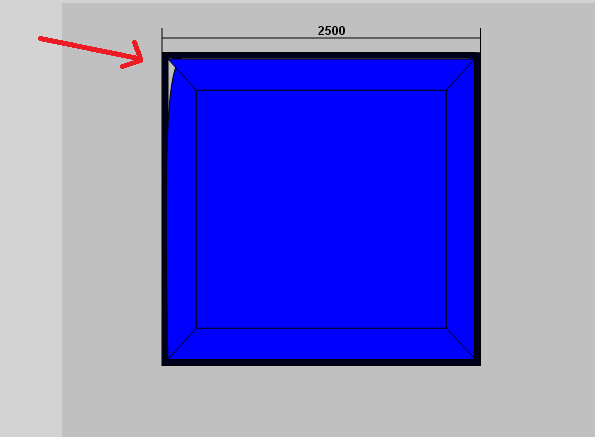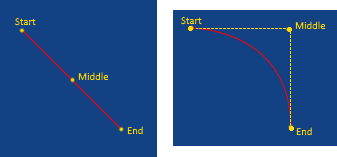# How does bezier curve drawing works?

## Recommended Posts

I was wondering how does bezier cureve drawing works. I know there is function bezierCurveTo(cpX, cpY, cpX2, cpY2, toX, toY) → {PIXI.Graphics}

and probably (cpX, cpY) is the start point, (cpX2, cpY2) is the middle control point and (toX, toY) is the ending point.

but I got something like:Which is the polygon I drew just before the bezierCurveTo() call.

Should I create first polygon and add fill/border to it or what do I do?

Are there some official Phaser examples for drawing bezier curves?

##### Share on other sites

Hi, maybe not what you exactly need, but best explanation of bezier curves and interpolation I have ever seen: https://www.youtube.com/watch?v=Zkx1aKv2z8o&t=15m0s

Also rest of the video is worth seeing it.

##### Share on other sites

Will check it later but its no use if it explains how are bezier curves drawn mathematically. I have reworked complete tutorial/asset in Unity3D which draws any type of bezier curve with 3 and 4 control points.

This one specifiaclly: http://catlikecoding.com/unity/tutorials/curves-and-splines/

I don't understand how phaser is drawing bezier curves. Is it like the lineTo() function, you first set up starting point with moveTo() and then call bezierCurveTo()?

Is it quadratic bezier with 3 control points?

##### Share on other sites

Ok this looks promising:

``````graphics = gameObject.add.graphics(0, 0);
graphics.lineStyle(1, borderColor, 1);
graphics.moveTo(400, 400);
graphics.bezierCurveTo(400, 400, 400, 500, 500, 500);``````

yes maybe it is like lineTo(). Needs moveTo() starting point.

##### Share on other sites

Haha, yes! bezier curve with (400, 400, 450, 450, 500, 500) will draw straight line. Because the control point, that "makes curvation" is on straight line between start and and point.

Change it to this: graphics.bezierCurveTo(400, 400, 500 , 400, 500, 500); to get nice arc:This is exactly explained on that video. Really recommend to see it. Bezier curve does not go through control points - it is only "attracted" to them. If you need to go through control points, then you need to use Catmull-Rom curve.

Edit: looking into source, Phaser / PIXI says:

``````/**
* Calculate the points for a bezier curve and then draws it.
*
* @method bezierCurveTo
* @param cpX {Number} Control point x
* @param cpY {Number} Control point y
* @param cpX2 {Number} Second Control point x
* @param cpY2 {Number} Second Control point y
* @param toX {Number} Destination point x
* @param toY {Number} Destination point y
* @return {Graphics}
*/
PIXI.Graphics.prototype.bezierCurveTo = function(cpX, cpY, cpX2, cpY2, toX, toY)``````

So, it is not quadratic, but cubic bezier curve and first point is given with current poision (moveTo() or end of previous drawing). Then you are passing only 2 control points and end point.